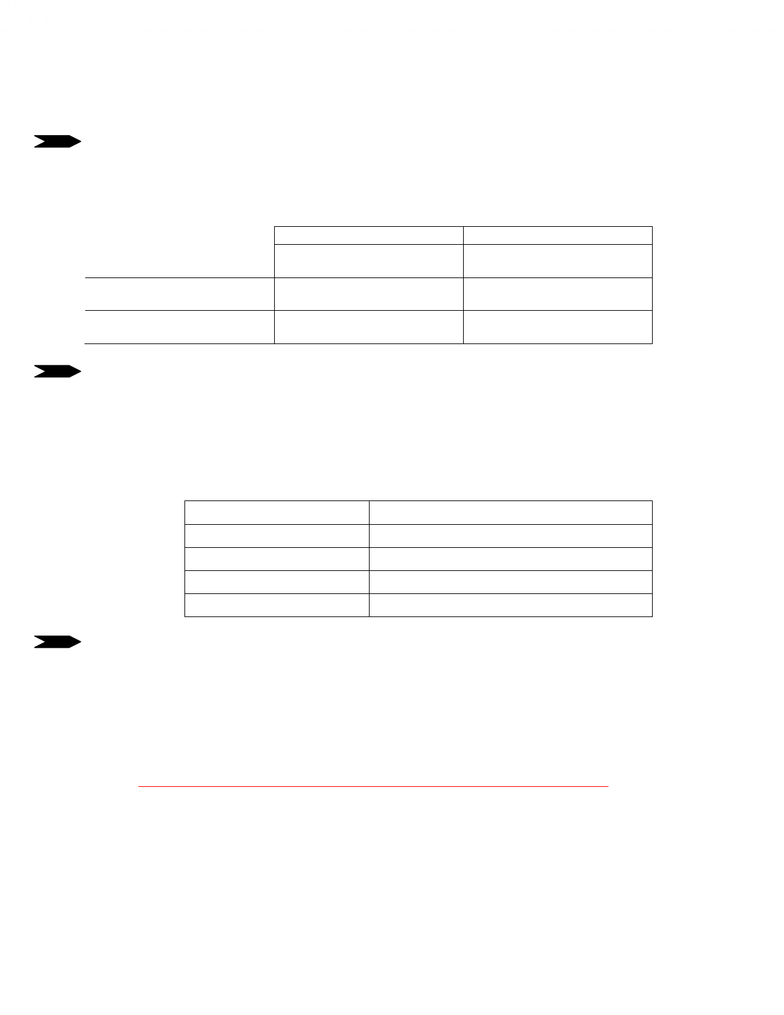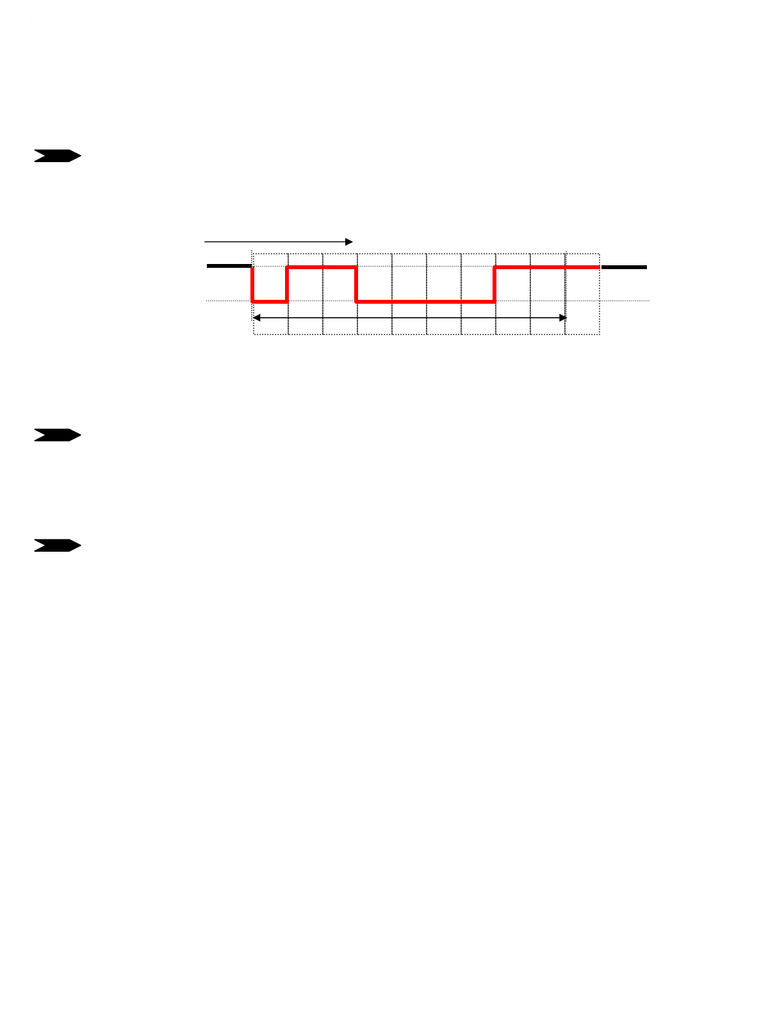# ECE385H1 Study Guide - Final Guide: University Of Toronto Faculty Of Applied Science And Engineering, Endianness, Parity Bit

54 views9 pages
School
UTSG
Department
Electrical & Computer Engineering
Course
ECE385H1
ProfessorNAME_______________________ or ID#____________________ (in case pages get detached)
UNIVERSITY OF TORONTO
FACULTY OF APPLIED SCIENCE AND ENGINEERING
MIDTERM EXAMINATION, FEBRUARY 2006
ECE243H1 S – COMPUTER ORGANIZATION
Exam Type: D
A. Moshovos, G. Steffan
This is a type D exam. You are allowed to use the course
textbook (any version), the lab manual (any version) and one
double-sided A4 (or 8.5”x11”) sheet with notes. Calculators
are not permitted.
Last Name (Print): __________________
First Name: ________________________
Student Number: ___________________
Marks Max.
Marks
A 30
B 30
C 30
D 10
Total 100
your time wisely as not all questions will require the same
amount of time. If you think that assumptions must be made to
answer a question, state them clearly. If there are multiple
possibilities, comment that there are, explain why and then
provide at least one possible answer and state the
corresponding assumptions.
There are 4 questions for a total of 100 marks. There are 1
double-sided
p
a
g
es in the exam.
Unlock document

This preview shows pages 1-3 of the document.
Unlock all 9 pages and 3 million more documents.NAME_______________________ or ID#____________________ (in case pages get detached)
1) Byte Order [6 marks] Assume that D0 is initialized to \$A1B2C3D4 before each of the
following three instruction pairs executes. Give the value (in hex) of the least-significant byte
of D1 after each of the following pairs of instructions have executed, for both little and big
endian machines (i.e., give only one value for each box):
Little Endian Big Endian
Move.l d0,\$1000
Move.l \$1000,d1
\$D4 \$D4
Move.w d0,\$1000
Move.b \$1001,d1
\$C3 \$D4
Move.l d0,\$1000
Move.w \$1002,d1
\$B2 \$D4
2) [6 marks] Assuming the following initialization:
a0 = \$00050000
d0 = \$00000120
Write the effective address (i.e., the memory address accessed) of the destination (in hex)
for each of the following, assuming the initialization above for each row (ie., all rows are
independent):
add.b d0,\$a0 \$a0 (the hex value)
3) [6 marks] The following 68K assembly sequence:
foo cmp.l d0, d1
bgt boo
sub.l d1, d0
boo sub.l d1, d0
implements one the following C statements:
(a) if (a > b) { b = b – a; } else {b = b – 2*a; }
(b) if (b > a) { b = b – a; } else {b = b – 2*a; }
(c) if (a == b) { b = a – b; } else { b = b – a; }
(d) if (a > b) { a= a – b; } else { a= b – a; }
(e) None of the above
Which one? Circle your answer. Figuring out which registers a and b map to is part of the
question.
Unlock document

This preview shows pages 1-3 of the document.
Unlock all 9 pages and 3 million more documents.NAME_______________________ or ID#____________________ (in case pages get detached)
4) [6 marks] Assume the following configuration of a serial connection: 20K BAUD (bit cells
per second), 8 bits including one parity bit, even parity, 1 stop bit.
a) If I transmit the ASCII encoding for 'C' (1000011 in binary), what bit sequence would I
expect to receive for the frame? I.e., fill in the signal diagram below between the idle periods,
clearly showing zeros and ones.
First is the START BIT. Then the 7 data bits for C starting from
bit 0, then the parity which is 1 so that all the number of ones
sent is even and finally the stop bit: 10 bits total: 0110000111
b) What is the effective data rate of this connection?
Each 7 bits of actual data needs an additional 3 bits during
transmission (start/stop/parity bits). Hence 20000 / 10 * 7 =
14000 bits/sec. Answers with 8 data bits will be considered
correct and so will be answers with 20K = 1024*20.
5) [6 marks] While we have not discussed this in our lectures, in C one might declare the
following array of pointers to integers:
int *array;
Assuming the array has already been initialized, give the assembly
instructions to implement the following statement:
x = x + *(array[i]);
Assume the following: x is in d0; i is in d1; ARRAY is a label defining the base address of
array; an int is a long word.
add.l d1, d1 ; i = 2 * i
add.l d1, d1 ; i = 4 * i
movea.l #ARRAY, a0 ; a0=base address
movea.l (a0),a0 ; a0 = array[i]
add.l (a0), d0 ; x = x + *array[i]
(idle) (idle)
0
1
Frame
Data
Wire:
Time
Unlock document

This preview shows pages 1-3 of the document.
Unlock all 9 pages and 3 million more documents.